# metrica: Prediction performance metrics.

## Introduction`metrica` is a compilation of more than 80 functions designed to quantitatively and visually evaluate the prediction performance of regression (continuous variables) and classification (categorical variables) point-forecast models (e.g. APSIM, DSSAT, DNDC, Supervised Machine Learning). `metrica` offers a toolbox with a wide spectrum of goodness of fit, error metrics, indices, and coefficients accounting for different aspects of the agreement between predicted and observed values, plus some basic visualization functions to assess models performance (e.g. confusion matrix, scatter with regression line; Bland-Altman plot) provided in customizable format (ggplot).

For supervised models, always keep in mind the concept of “cross-validation” since predicted values should ideally come from out-of-bag samples (unseen by training sets) to avoid overestimation of the prediction performance.

Vignettes

## Functions

For regression models, it includes 4 plotting functions (scatter, tiles, density, & Bland-Altman plots), and 48 prediction performance scores including error metrics (MBE, MAE, RAE, RMAE, MAPE, SMAPE, MSE, RMSE, RRMSE, RSR, PBE, iqRMSE), error decomposition (MLA, MLP, PLA, PLP, PAB, PPB, SB, SDSD, LCS, Ub, Uc, Ue), model efficiency (NSE, E1, Erel, KGE), indices of agreement (d, d1, d1r, RAC, AC, lambda), goodness of fit (r, R2, RSS, TSS, RSE), adjusted correlation coefficients (CCC, Xa, distance correlation-dcorr-, maximal information coefficient -MIC-), variability (uSD, var_u), and symmetric regression coefficients (B0_sma, B1_sma). Specifically for time-series predictions, `metrica` also includes the Mean Absolute Scaled Error (MASE).

For classification (binomial and multinomial) tasks, it includes a function to visualize the confusion matrix using ggplot2, and 27 functions of prediction scores including: accuracy, error rate, precision (predictive positive value -ppv-), recall (or true positive rate-TPR-), specificity (or true negative rate-TNR-, or selectivity), balanced accuracy (balacc), F-score (fscore), adjusted F-score (agf), G-mean (gmean), Bookmaker Informedness (bmi, a.k.a. Youden’s J-index -jindex-), Markedness (deltaP, or mk), Matthews Correlation Coefficient (mcc, a.k.a. phi-coefficient), Cohen’s Kappa (khat), negative predictive value (npv), positive and negative likelihood ratios (posLr, negLr), diagnostic odds ratio (dor), prevalence (preval), prevalence threshold (preval_t), critical success index (csi, a.k.a. threat score or Jaccard Index -jaccardindex-), false positive rate (FPR), false negative rate (FNR), false detection rate (FDR), false omission rate (FOR), and area under the ROC curve (AUC_roc).

`metrica` also offers a function () that allows users to run all prediction performance scores at once. The user just needs to specify the type of model (“regression” or “classification”).

For more details visit the vignettes https://adriancorrendo.github.io/metrica/.

## Using the functions

There are two basic arguments common to all `metrica` functions: (i) `obs`(Oi; observed, a.k.a. actual, measured, truth, target, label), and (ii) `pred` (Pi; predicted, a.k.a. simulated, fitted, modeled, estimate) values.

Optional arguments include `data` that allows to call an existing data frame containing both observed and predicted vectors, and `tidy`, which controls the type of output as a list (tidy = FALSE) or as a data.frame (tidy = TRUE).

For regression, some specific functions for regression also require to define the axis `orientation`. For example, the slope of the symmetric linear regression describing the bivariate scatter (SMA).

For binary classification (two classes), functions also require to check the `pos_level` arg., which indicates the alphanumeric order of the “positive level”. Normally, the most common binary denominations are c(0,1), c(“Negative”, “Positive”), c(“FALSE”, “TRUE”), so the default pos_level = 2 (1, “Positive”, “TRUE”). However, other cases are also possible, such as c(“Crop”, “NoCrop”) for which the user needs to specify pos_level = 1.

For multiclass classification tasks, some functions present the `atom` arg. (logical TRUE / FALSE), which controls the output to be an overall average estimate across all classes, or a class-wise estimate. For example, user might be interested in obtaining estimates of precision and recall for each possible class of the prediction.

## 1. Installation

You can install the CRAN version of `metrica` with:

``install.packages("metrica")``

You can install the development version from GitHub with:

``````# install.packages("devtools")

## 2. Native datasets

The metrica package comes with four example datasets of continuous variables (regression) from the APSIM software:

1. `wheat`. 137 data-points of wheat grain N (grams per squared meter)
2. `barley`. 69 data-points of barley grain number (x1000 grains per squared meter)
3. `sorghum`. 36 data-points of sorghum grain number (x1000 grains per squared meter)
4. `chickpea`. 39 data-points of chickpea aboveground dry mass (kg per hectare)

These data correspond to the latest, up-to-date, documentation and validation of version number 2020.03.27.4956. Data available at: https://doi.org/10.7910/DVN/EJS4M0. Further details can be found at the official APSIM Next Generation website: https://APSIMnextgeneration.netlify.app/modeldocumentation

In addition, `metrica` also provides two native examples for categorical variables (classification):

1. `land_cover` is a binary dataset of land cover using satellite images obtained in 2022 over a small region in Kansas (USA). Values equal to 1 are associated to vegetation, and values equal to 0 represent other type of land cover. Observed values come from human visualization, while predicted values were obtained with a Random Forest classifier.

2. `maize_phenology` is a data set of maize/corn (Zea mays L.) phenology (crop development stage) collected in Kansas (USA) during 2018. The data includes 16 different phenology stages. Observed values were obtained via human visualization, while predicted values were obtained with a Random Forest classifier.

Any of the above-mentioned data sets can be called with `metrica::name_of_dataset`, for example:

``````metrica::wheat
metrica::land_cover``````

## 3. Example Code

### Libraries

``````library(metrica)
library(dplyr)
library(purrr)
library(ggplot2)
library(tidyr)``````

This is a basic example which shows you the core regression and classification functions of metrica:

## 3.1. REGRESSION

``````# 1. A. Create a random dataset
# Set seed for reproducibility
set.seed(1)
# Create a random vector (X) with 100 values
X <- rnorm(n = 100, mean = 0, sd = 10)
# Create a second vector (Y) with 100 values by adding error with respect
# to the first vector (X).
Y <- X + rnorm(n=100, mean = 0, sd = 3)
# Merge vectors in a data frame, rename them as synonyms of observed (measured) and predicted (simulated)
example.data <- data.frame(measured = X, simulated = Y)

# 1. B. Or call native example datasets

example.data <- barley %>%  # or 'wheat', 'sorghum', or 'chickpea'
# 1.b. create columns as synonyms of observed (measured) and predicted (simulated)
mutate(measured = obs, simulated = pred)  ``````

### 3.1.1.1. Create a customizable scatter plot with PO orientation

``````
barley.scat.plot <-
metrica::scatter_plot(data = example.data,
obs = measured,
pred = simulated,
orientation = "PO",
print_eq = TRUE,
position_eq = c(x=24, y =8),
# Optional arguments to customize the plot
shape_type = 21,
shape_color = "steelblue",
shape_size = 3,
regline_type = "F1",
regline_color = "#9e0059",
regline_size = 2)+
# Customize axis breaks
scale_y_continuous(breaks = seq(0,30, by = 5))+
scale_x_continuous(breaks = seq(0,30, by = 5))

barley.scat.plot``````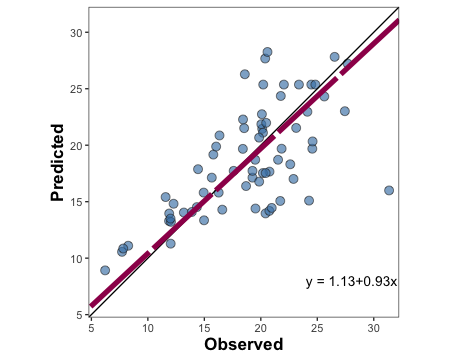``````
# Alternative using vectors instead of dataframe
#metrica::scatter_plot(obs = example.data\$obs, pred = example.data\$pred)``````

### 3.1.1.2. Create tiles plot with OP orientation

``````barley.tiles.plot <-
tiles_plot(data = example.data,
obs = measured,
pred = simulated,
bins = 10,
orientation = "PO",
colors = c(low = "pink", high = "steelblue"))

barley.tiles.plot``````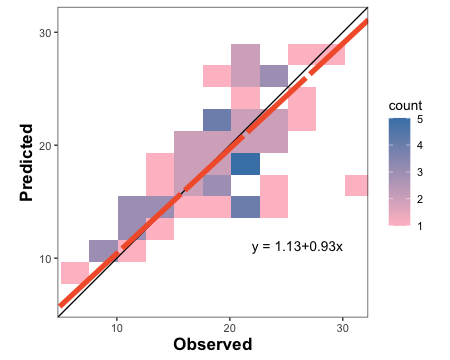### 3.1.1.3. Create a density plot with OP orientation

``````barley.density.plot <-
metrica::density_plot(data = example.data,
obs = measured, pred = simulated,
n = 5,
orientation = "PO",
colors = c(low = "white", high = "steelblue") )+
theme(legend.position = "none")

barley.density.plot``````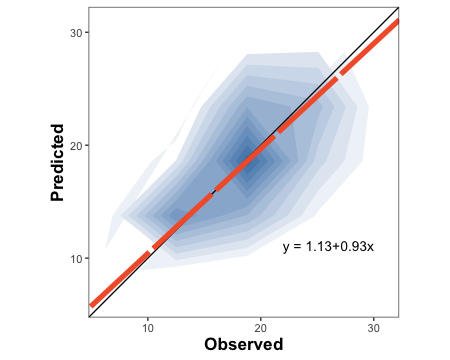### 3.1.1.4. Create a Bland-Altman plot

``````barley.ba.plot <- metrica::bland_altman_plot(data = example.data,
obs = measured, pred = simulated)

barley.ba.plot``````### 3.1.2.2. Single estimates

``````# a. Estimate coefficient of determination (R2)

metrica::R2(data = example.data, obs = measured, pred = simulated)
#> \$R2
#>  0.4512998

# b. Estimate root mean squared error (RMSE)
metrica::RMSE(data = example.data, obs = measured, pred = simulated)
#> \$RMSE
#>  3.986028

# c. Estimate mean bias error (MBE)
metrica::MBE(data = example.data, obs = measured, pred = simulated)
#> \$MBE
#>  0.207378

# c. Estimate index of agreement (d)
metrica::d(data = example.data, obs = measured, pred = simulated)
#> \$d
#>  0.8191397

# e. Estimate SMA regression intercept (B0)
metrica::B0_sma(data = example.data, obs = measured, pred = simulated, tidy = TRUE)
#>         B0
#> 1 1.128274

# f. Estimate SMA regression slope (B1)
metrica::B1_sma(data = example.data, obs = measured, pred = simulated)
#> \$B1
#>  0.9288715``````

### 3.1.2.2. Metrics Summary

``````
metrics.sum <- metrics_summary(data = example.data,
obs = measured, pred = simulated,
type = "regression")
# Print first 15
#>    Metric      Score
#> 1      B0  1.1282743
#> 2      B1  0.9288715
#> 3       r  0.6717885
#> 4      R2  0.4512998
#> 5      Xa  0.9963915
#> 6     CCC  0.6693644
#> 7     MAE  3.0595501
#> 8    RMAE  0.1629325
#> 9    MAPE 16.8112673
#> 10  SMAPE 16.7848032
#> 11    RAE  0.7639151
#> 12    RSE  0.6164605
#> 13    MBE  0.2073780
#> 14    PBE  1.1043657
#> 15    PAB  0.2706729

# Optional wrangling (WIDE)
metrics.sum.wide <- metrics.sum %>%
tidyr::pivot_wider(tidyr::everything(),
names_from = "Metric",
values_from = "Score")

metrics.sum.wide
#> # A tibble: 1 × 45
#>      B0    B1     r    R2    Xa   CCC   MAE  RMAE  MAPE SMAPE   RAE   RSE   MBE
#>   <dbl> <dbl> <dbl> <dbl> <dbl> <dbl> <dbl> <dbl> <dbl> <dbl> <dbl> <dbl> <dbl>
#> 1  1.13 0.929 0.672 0.451 0.996 0.669  3.06 0.163  16.8  16.8 0.764 0.616 0.207
#> # … with 32 more variables: PBE <dbl>, PAB <dbl>, PPB <dbl>, MSE <dbl>,
#> #   RMSE <dbl>, RRMSE <dbl>, RSR <dbl>, iqRMSE <dbl>, MLA <dbl>, MLP <dbl>,
#> #   RMLA <dbl>, RMLP <dbl>, SB <dbl>, SDSD <dbl>, LCS <dbl>, PLA <dbl>,
#> #   PLP <dbl>, Ue <dbl>, Uc <dbl>, Ub <dbl>, NSE <dbl>, E1 <dbl>, Erel <dbl>,
#> #   KGE <dbl>, d <dbl>, d1 <dbl>, d1r <dbl>, RAC <dbl>, AC <dbl>, lambda <dbl>,
#> #   dcorr <dbl>, MIC <dbl>``````

### 3.1.3.1. Nested data

``````# a. Create nested df with the native examples
nested.examples <- bind_rows(list(wheat = metrica::wheat,
barley = metrica::barley,
sorghum = metrica::sorghum,
chickpea = metrica::chickpea),
.id = "id") %>%
dplyr::group_by(id) %>% tidyr::nest() %>% dplyr::ungroup()

#> # A tibble: 4 × 2
#> # Groups:   id 
#>   id       data
#>   <chr>    <list>
#> 1 barley   <tibble [69 × 2]>
#> 2 chickpea <tibble [39 × 2]>
#> 3 sorghum  <tibble [36 × 2]>
#> 4 wheat    <tibble [137 × 2]>

# b. Run
multiple.sum <- nested.examples %>%
# Store metrics in new.column "performance"
mutate(performance = map(
data, ~metrica::metrics_summary(data=., obs = obs, pred = pred,
type = "regression")))

#> # A tibble: 4 × 3
#>   id       data               performance
#>   <chr>    <list>             <list>
#> 1 wheat    <tibble [137 × 2]> <df [45 × 2]>
#> 2 barley   <tibble [69 × 2]>  <df [45 × 2]>
#> 3 sorghum  <tibble [36 × 2]>  <df [45 × 2]>
#> 4 chickpea <tibble [39 × 2]>  <df [45 × 2]>``````

### 3.1.3.2. Non-nested data

``````non_nested_summary <- nested.examples %>% unnest(cols = "data") %>%
group_by(id) %>%
summarise(metrics_summary(obs = obs, pred = pred, type = "regression")) %>%
dplyr::arrange(Metric)

#> # A tibble: 6 × 3
#> # Groups:   id 
#>   id       Metric    Score
#>   <chr>    <chr>     <dbl>
#> 1 barley   AC       0.253
#> 2 chickpea AC       0.434
#> 3 sorghum  AC       0.0889
#> 4 wheat    AC       0.842
#> 5 barley   B0       1.13
#> 6 chickpea B0     -99.0``````
``````df <- metrica::wheat

# Create list of selected metrics
selected.metrics <- c("MAE","RMSE", "RRMSE", "R2", "NSE", "KGE", "PLA", "PLP")

df <- metrica::wheat
# Create the plot
plot <- metrica::scatter_plot(data = df,
obs = obs, pred = pred,
# Activate print_metrics arg.
print_metrics = TRUE,
# Indicate metrics list
metrics_list = selected.metrics,
# Customize metrics position
position_metrics = c(x = 16 , y = 9),
# Customize equation position
position_eq = c(x = 16.2, y = 9.5))

plot``````## 3.1. CLASSIFICATION

### Example datasets

``````binomial_case <- data.frame(labels = sample(c("Pos","Neg"), 100, replace = TRUE),
predictions = sample(c("Pos","Neg"), 100, replace = TRUE)) %>%
mutate(predictions = as.factor(predictions), labels = as.factor(labels))

multinomial_case <- data.frame(labels = sample(c("Red","Green", "Blue"), 100, replace = TRUE),
predictions = sample(c("Red","Green", "Blue"), 100, replace = TRUE) ) %>%
mutate(predictions = as.factor(predictions), labels = as.factor(labels))``````

### 3.1.1.1. Binary

``````# a. Print
binomial_case %>% confusion_matrix(obs = labels, pred = predictions,
plot = FALSE, colors = c(low="#f9dbbd" , high="#735d78"),
unit = "count")
#>          OBSERVED
#> PREDICTED Neg Pos
#>       Neg  24  24
#>       Pos  21  31

# b. Plot
binomial_case %>% confusion_matrix(obs = labels, pred = predictions,
plot = TRUE, colors = c(low="#f9dbbd" , high="#735d78"),
unit = "count", print_metrics = TRUE)``````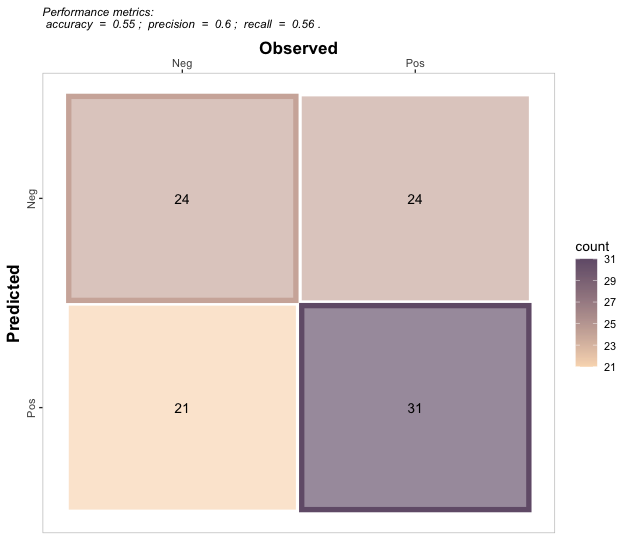### 3.1.1.2. Multiclass

``````# a. Print
multinomial_case %>% confusion_matrix(obs = labels,
pred = predictions,
plot = FALSE, colors = c(low="#f9dbbd" , high="#735d78"),
unit = "count")
#>          OBSERVED
#> PREDICTED Blue Green Red
#>     Blue     9    11   9
#>     Green   11    12  11
#>     Red     13    13  11

# b. Plot
multinomial_case %>% confusion_matrix(obs = labels,
pred = predictions,
plot = TRUE, colors = c(low="#d3dbbd" , high="#885f78"),
unit = "count", print_metrics = TRUE)``````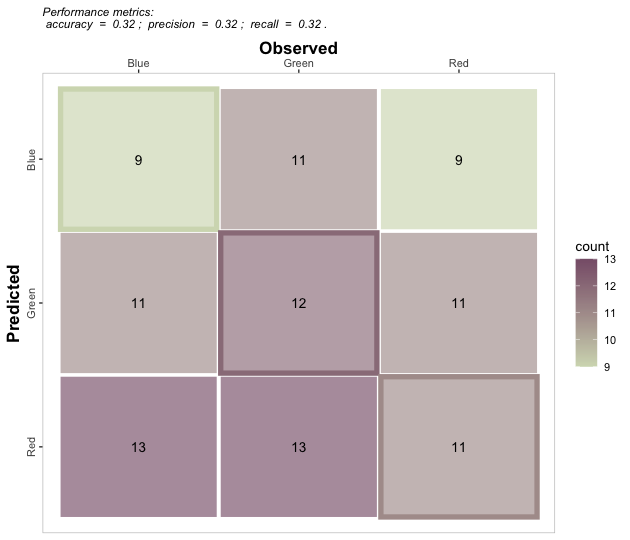### 3.1.1.1. Single dataset

``````# Get classification metrics one by one
binomial_case %>% accuracy(data = ., obs = labels, pred = predictions, tidy=TRUE)
#>   accuracy
#> 1     0.55
binomial_case %>% error_rate(data = ., obs = labels, pred = predictions, tidy=TRUE)
#>   error_rate
#> 1       0.45
binomial_case %>% precision(data = ., obs = labels, pred = predictions, tidy=TRUE)
#>   precision
#> 1 0.5961538
binomial_case %>% recall(data = ., obs = labels, pred = predictions, atom = F, tidy=TRUE)
#>      recall
#> 1 0.5636364
binomial_case %>% specificity(data = ., obs = labels, pred = predictions, tidy=TRUE)
#>        spec
#> 1 0.5333333
binomial_case %>% balacc(data = ., obs = labels, pred = predictions, tidy=TRUE)
#>      balacc
#> 1 0.5484848
binomial_case %>% fscore(data = ., obs = labels, pred = predictions, tidy=TRUE)
#>      fscore
#> 1 0.5794393
binomial_case %>% agf(data = ., obs = labels, pred = predictions, tidy=TRUE)
#>         agf
#> 1 0.5795213
binomial_case %>% gmean(data = ., obs = labels, pred = predictions, tidy=TRUE)
#>       gmean
#> 1 0.5482755
binomial_case %>% khat(data = ., obs = labels, pred = predictions, tidy=TRUE)
#>         khat
#> 1 0.09638554
binomial_case %>% mcc(data = ., obs = labels, pred = predictions, tidy=TRUE)
#>          mcc
#> 1 0.09656091
binomial_case %>% fmi(data = ., obs = labels, pred = predictions, tidy=TRUE)
#>         fmi
#> 1 0.5796671
binomial_case %>% posLr(data = ., obs = labels, pred = predictions, tidy=TRUE)
#>      posLr
#> 1 1.207792
binomial_case %>% negLr(data = ., obs = labels, pred = predictions, tidy=TRUE)
#>       negLr
#> 1 0.8181818
binomial_case %>% dor(data = ., obs = labels, pred = predictions, tidy=TRUE)
#>       dor
#> 1 1.47619

# Get all at once with metrics_summary()
binomial_case %>% metrics_summary(data = ., obs = labels, pred = predictions, type = "classification")
#>         Metric      Score
#> 1     accuracy 0.55000000
#> 2   error_rate 0.45000000
#> 3    precision 0.59615385
#> 4       recall 0.56363636
#> 5  specificity 0.53333333
#> 6       balacc 0.54848485
#> 7       fscore 0.57943925
#> 8          agf 0.57952126
#> 9        gmean 0.54827553
#> 10        khat 0.09638554
#> 11         mcc 0.09656091
#> 12         fmi 0.57966713
#> 13         bmi 0.09696970
#> 14         csi 0.40789474
#> 15      deltap 0.09615385
#> 16       posLr 1.20779221
#> 17       negLr 0.81818182
#> 18         dor 1.47619048
#> 19         npv 0.50000000
#> 20         FPR 0.46666667
#> 21         FNR 0.43636364
#> 22         FDR 0.40384615
#> 23         FOR 0.50000000
#> 24      preval 0.55000000
#> 25    preval_t 0.49309260
#> 26     AUC_roc 0.54848485

# Multinomial
multinomial_case %>% metrics_summary(data = ., obs = labels, pred = predictions, type = "classification")
#> Warning in metrica::fscore(data = ~., obs = ~labels, pred = ~predictions, : For
#> multiclass cases, the fscore should be estimated at a class level. Please,
#> consider using `atom = TRUE`
#> Warning in metrica::agf(data = ~., obs = ~labels, pred = ~predictions,
#> pos_level = pos_level): For multiclass cases, the agf should be estimated at a
#> class level. Please, consider using `atom = TRUE`
#> Warning in metrica::fmi(data = ~., obs = ~labels, pred = ~predictions,
#> pos_level = pos_level): The Fowlkes-Mallows Index is not available for
#> multiclass cases. The result has been recorded as NaN.
#> Warning in metrica::preval(data = ~., obs = ~labels, pred = ~predictions, : For
#> multiclass cases, prevalence should be estimated at a class level. A NaN has
#> been recorded as the result. Please, use `atom = TRUE`
#> Warning in metrica::preval_t(data = ~., obs = ~labels, pred = ~predictions, : For multiclass cases, prevalence threshold should be estimated at a class level.
#>       A NaN has been recorded as the result. Please, use `atom = TRUE`.
#>         Metric       Score
#> 1     accuracy  0.32000000
#> 2   error_rate  0.68000000
#> 3    precision  0.32019443
#> 4       recall  0.32029977
#> 5  specificity  0.66031031
#> 6       balacc  0.49030504
#> 7       fscore  0.32024709
#> 8          agf  0.32024710
#> 9        gmean  0.45988829
#> 10        khat -0.01918465
#> 11         mcc -0.01926552
#> 12         fmi         NaN
#> 13         bmi -0.01938991
#> 14         csi  0.13793860
#> 15      deltap -0.01951385
#> 16       posLr  0.94291874
#> 17       negLr  1.02936485
#> 18         dor  0.91601996
#> 19         npv  0.66029172
#> 20         FPR  0.33968969
#> 21         FNR  0.67970023
#> 22         FDR  0.67980557
#> 23         FOR  0.33970828
#> 24      preval         NaN
#> 25    preval_t         NaN
#> 26     AUC_roc  0.49030504

# Get a selected list at once with metrics_summary()
selected_class_metrics <- c("accuracy", "recall", "fscore")

# Binary
binomial_case %>% metrics_summary(data = ., obs = labels, pred = predictions, type = "classification",
metrics_list = selected_class_metrics)
#>     Metric     Score
#> 1 accuracy 0.5500000
#> 2   recall 0.5636364
#> 3   fscore 0.5794393

# Multiclass
multinomial_case %>% metrics_summary(data = ., obs = labels, pred = predictions, type = "classification",
metrics_list = selected_class_metrics)
#> Warning in metrica::fscore(data = ~., obs = ~labels, pred = ~predictions, : For
#> multiclass cases, the fscore should be estimated at a class level. Please,
#> consider using `atom = TRUE`
#> Warning in metrica::agf(data = ~., obs = ~labels, pred = ~predictions,
#> pos_level = pos_level): For multiclass cases, the agf should be estimated at a
#> class level. Please, consider using `atom = TRUE`
#> Warning in metrica::fmi(data = ~., obs = ~labels, pred = ~predictions,
#> pos_level = pos_level): The Fowlkes-Mallows Index is not available for
#> multiclass cases. The result has been recorded as NaN.
#> Warning in metrica::preval(data = ~., obs = ~labels, pred = ~predictions, : For
#> multiclass cases, prevalence should be estimated at a class level. A NaN has
#> been recorded as the result. Please, use `atom = TRUE`
#> Warning in metrica::preval_t(data = ~., obs = ~labels, pred = ~predictions, : For multiclass cases, prevalence threshold should be estimated at a class level.
#>       A NaN has been recorded as the result. Please, use `atom = TRUE`.
#>     Metric     Score
#> 1 accuracy 0.3200000
#> 2   recall 0.3202998
#> 3   fscore 0.3202471``````
``````
multinomial_case %>% accuracy(data = ., obs = labels, pred = predictions, tidy=TRUE)
#>   accuracy
#> 1     0.32
multinomial_case %>% error_rate(data = ., obs = labels, pred = predictions, tidy=TRUE)
#>   error_rate
#> 1       0.68
multinomial_case %>% precision(data = ., obs = labels, pred = predictions, tidy=TRUE)
#>   precision
#> 1 0.3201944
multinomial_case %>% recall(data = ., obs = labels, pred = predictions, atom = F, tidy=TRUE)
#>      recall
#> 1 0.3202998
multinomial_case %>% specificity(data = ., obs = labels, pred = predictions, tidy=TRUE)
#>        spec
#> 1 0.6603103
multinomial_case %>% balacc(data = ., obs = labels, pred = predictions, tidy=TRUE)
#>     balacc
#> 1 0.490305
multinomial_case %>% fscore(data = ., obs = labels, pred = predictions, tidy=TRUE)
#> Warning in fscore(data = ., obs = labels, pred = predictions, tidy = TRUE): For
#> multiclass cases, the fscore should be estimated at a class level. Please,
#> consider using `atom = TRUE`
#>      fscore
#> 1 0.3202471
multinomial_case %>% agf(data = ., obs = labels, pred = predictions, tidy=TRUE)
#> Warning in agf(data = ., obs = labels, pred = predictions, tidy = TRUE): For
#> multiclass cases, the agf should be estimated at a class level. Please,
#> consider using `atom = TRUE`
#>         agf
#> 1 0.3202471
multinomial_case %>% gmean(data = ., obs = labels, pred = predictions, tidy=TRUE)
#>       gmean
#> 1 0.4598883
multinomial_case %>% khat(data = ., obs = labels, pred = predictions, tidy=TRUE)
#>          khat
#> 1 -0.01918465
multinomial_case %>% mcc(data = ., obs = labels, pred = predictions, tidy=TRUE)
#>           mcc
#> 1 -0.01926552
multinomial_case %>% fmi(data = ., obs = labels, pred = predictions, tidy=TRUE)
#> Warning in fmi(data = ., obs = labels, pred = predictions, tidy = TRUE): The
#> Fowlkes-Mallows Index is not available for multiclass cases. The result has
#> been recorded as NaN.
#>   fmi
#> 1 NaN
multinomial_case %>% posLr(data = ., obs = labels, pred = predictions, tidy=TRUE)
#>       posLr
#> 1 0.9429187
multinomial_case %>% negLr(data = ., obs = labels, pred = predictions, tidy=TRUE)
#>      negLr
#> 1 1.029365
multinomial_case %>% dor(data = ., obs = labels, pred = predictions, tidy=TRUE)
#>       dor
#> 1 0.91602
multinomial_case %>% deltap(data = ., obs = labels, pred = predictions, tidy=TRUE)
#>        deltap
#> 1 -0.01951385
multinomial_case %>% csi(data = ., obs = labels, pred = predictions, tidy=TRUE)
#>         csi
#> 1 0.1379386
multinomial_case %>% FPR(data = ., obs = labels, pred = predictions, tidy=TRUE)
#>         FPR
#> 1 0.3396897
multinomial_case %>% FNR(data = ., obs = labels, pred = predictions, tidy=TRUE)
#>         FNR
#> 1 0.6797002
multinomial_case %>% FDR(data = ., obs = labels, pred = predictions, tidy=TRUE)
#>         FDR
#> 1 0.6798056
multinomial_case %>% FOR(data = ., obs = labels, pred = predictions, tidy=TRUE)
#>         FOR
#> 1 0.3397083
multinomial_case %>% preval(data = ., obs = labels, pred = predictions, tidy=TRUE)
#> Warning in preval(data = ., obs = labels, pred = predictions, tidy = TRUE): For
#> multiclass cases, prevalence should be estimated at a class level. A NaN has
#> been recorded as the result. Please, use `atom = TRUE`
#>   prev
#> 1  NaN
multinomial_case %>% preval_t(data = ., obs = labels, pred = predictions, tidy=TRUE)
#> Warning in preval_t(data = ., obs = labels, pred = predictions, tidy = TRUE): For multiclass cases, prevalence threshold should be estimated at a class level.
#>       A NaN has been recorded as the result. Please, use `atom = TRUE`.
#>   preval_t
#> 1      NaN
multinomial_case %>% AUC_roc(data = ., obs = labels, pred = predictions, tidy=TRUE)
#>    AUC_roc
#> 1 0.490305``````

## 5. Contributing to our package

Thank you for considering contributing to our open-source project. Although we are not directly funded to maintain `metrica`, we care about reproducible science, like you. Thus, all contributions are more than welcome!

There are multiple ways you can contribute to `metrica` such as asking questions, propose ideas, report bugs, improve the vignettes & documentation of functions, as well as contributing with code, of course.

For comments, suggestions, and bug reports, we highly encourage to use our GitHub issues section.

To improve the documentation and contribute with code, we encourage to fork the repo and use pull requests to contribute code.

## 6. Code of Conduct

Please note that the metrica project is released with a Contributor Code of Conduct. By contributing to this project, you agree to abide by its terms.